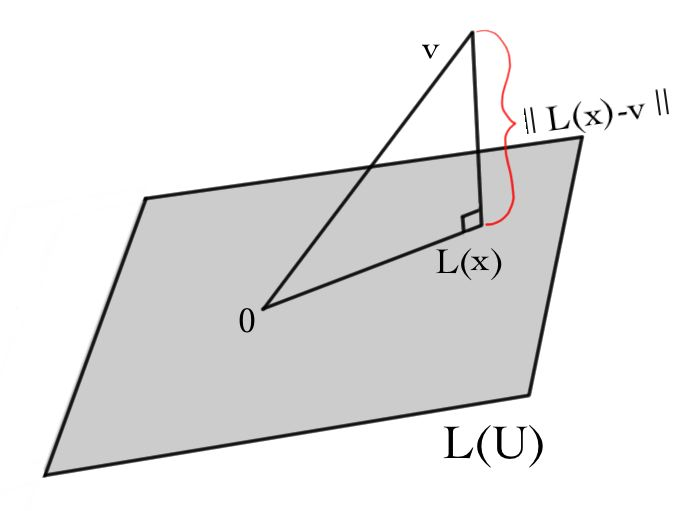# 17: Least Squares and Singular Values

$$\newcommand{\vecs}{\overset { \rightharpoonup} {\mathbf{#1}} }$$ $$\newcommand{\vecd}{\overset{-\!-\!\rightharpoonup}{\vphantom{a}\smash {#1}}}$$$$\newcommand{\id}{\mathrm{id}}$$ $$\newcommand{\Span}{\mathrm{span}}$$ $$\newcommand{\kernel}{\mathrm{null}\,}$$ $$\newcommand{\range}{\mathrm{range}\,}$$ $$\newcommand{\RealPart}{\mathrm{Re}}$$ $$\newcommand{\ImaginaryPart}{\mathrm{Im}}$$ $$\newcommand{\Argument}{\mathrm{Arg}}$$ $$\newcommand{\norm}{\| #1 \|}$$ $$\newcommand{\inner}{\langle #1, #2 \rangle}$$ $$\newcommand{\Span}{\mathrm{span}}$$ $$\newcommand{\id}{\mathrm{id}}$$ $$\newcommand{\Span}{\mathrm{span}}$$ $$\newcommand{\kernel}{\mathrm{null}\,}$$ $$\newcommand{\range}{\mathrm{range}\,}$$ $$\newcommand{\RealPart}{\mathrm{Re}}$$ $$\newcommand{\ImaginaryPart}{\mathrm{Im}}$$ $$\newcommand{\Argument}{\mathrm{Arg}}$$ $$\newcommand{\norm}{\| #1 \|}$$ $$\newcommand{\inner}{\langle #1, #2 \rangle}$$ $$\newcommand{\Span}{\mathrm{span}}$$$$\newcommand{\AA}{\unicode[.8,0]{x212B}}$$

Consider the linear system $$L(x)=v$$, where $$L \colon U\stackrel{\textit{linear}}{-\!\!\!-\!\!\!\longrightarrow}W$$, and $$v\in W$$ is given. As we have seen, this system may have no solutions, a unique solution, or a space of solutions. But if $$v$$ is not in the range of $$L$$, in pictures:there will never be any solutions for $$L(x)=v$$. However, for many applications we do not need an exact solution of the system; instead, we try to find the best approximation possible.

$\textit{"My work always tried to unite the Truth with the Beautiful, but when I had to choose one or the other, I usually chose the} \textit{Beautiful.''--Hermann Weyl.}$

If the vector space $$W$$ has a notion of lengths of vectors, we can try to find $$x$$ that minimizes $$||L(x)-v||$$:This method has many applications, such as when trying to fit a (perhaps linear) function to a "noisy'' set of observations. For example, suppose we measured the position of a bicycle on a racetrack once every five seconds. Our observations won't be exact, but so long as the observations are right on average, we can figure out a best-possible linear function of position of the bicycle in terms of time.

Suppose $$M$$ is the matrix for $$L$$ in some bases for $$U$$ and $$W$$, and $$v$$ and $$x$$ are given by column vectors $$V$$ and $$X$$ in these bases. Then we need to approximate

$MX-V\approx 0\, .$

Note that if $$\dim U=n$$ and $$\dim W=m$$ then $$M$$ can be represented by an $$m\times n$$ matrix and $$x$$ and $$v$$ as vectors in $$\Re^{n}$$ and $$\Re^{m}$$, respectively. Thus, we can write $$W=L(U)\oplus L(U)^{\perp}$$. Then we can uniquely write $$v=v^{\parallel} + v^{\perp}$$, with $$v^{\parallel} \in L(U)$$ and $$v^{\perp} \in L(U)^{\perp}$$.

Thus we should solve $$L(u)=v^{\parallel}$$. In components, $$v^{\perp}$$ is just $$V-MX$$, and is the part we will eventually wish to minimize.

In terms of $$M$$, recall that $$L(V)$$ is spanned by the columns of $$M$$. (In the standard basis, the columns of $$M$$ are $$Me_{1}$$, $$\ldots$$, $$Me_{n}$$.) Then $$v^{\perp}$$ must be perpendicular to the columns of $$M$$. $$\textit{i.e.}$$, $$M^{T}(V-MX)=0$$, or

$M^{T}MX = M^{T}V.$

Solutions of $$M^{T}MX = M^{T}V$$ for $$X$$ are called $$\textit{least squares}$$ solutions to $$MX=V$$. Notice that any solution $$X$$ to $$MX=V$$ is a least squares solution. However, the converse is often false. In fact, the equation $$MX=V$$ may have no solutions at all, but still have least squares solutions to $$M^{T}MX = M^{T}V$$.

Observe that since $$M$$ is an $$m\times n$$ matrix, then $$M^{T}$$ is an $$n\times m$$ matrix. Then $$M^{T}M$$ is an $$n\times n$$ matrix, and is symmetric, since $$(M^{T}M)^{T}=M^{T}M$$. Then, for any vector $$X$$, we can evaluate $$X^{T}M^{T}MX$$ to obtain a number. This is a very nice number, though! It is just the length $$|MX|^{2} = (MX)^{T}(MX)=X^{T}M^{T}MX$$.

Now suppose that $$\ker L=\{0\}$$, so that the only solution to $$MX=0$$ is $$X=0$$. (This need not mean that $$M$$ is invertible because $$M$$ is an $$n\times m$$ matrix, so not necessarily square.) However the square matrix $$M^{T}M$$ $$\textit{is}$$ invertible. To see this, suppose there was a vector $$X$$ such that $$M^{T} M X=0$$. Then it would follow that $$X^{T} M^{T} M X = |M X|^{2}=0$$. In other words the vector $$MX$$ would have zero length, so could only be the zero vector. But we are assuming that $$\ker L=\{0\}$$ so $$MX=0$$ implies $$X=0$$. Thus the kernel of $$M^{T}M$$ is $$\{0\}$$ so this matrix is invertible. So, in this case, the least squares solution (the $$X$$ that solves $$M^{T}MX=MV$$) is unique, and is equal to

$X = (M^{T}M)^{-1}M^{T}V.$

In a nutshell, this is the least squares method:

1. Compute $$M^{T}M$$ and $$M^{T}V$$.
2. Solve $$(M^{T}M)X=M^{T}V$$ by Gaussian elimination.

Example 134

Captain Conundrum falls off of the leaning tower of Pisa and makes three (rather shaky) measurements of his velocity at three different times.

$\begin{array}{r|r} t s & v m/s \\ \hline 1 & 11 \\ 2 & 19 \\ 3 & 31\end{array}$

Having taken some calculus, he believes that his data are best approximated by a straight line

$v = at+b.$

Then he should find $$a$$ and $$b$$ to best fit the data.

\begin{eqnarray*}
11 &=& a\cdot 1 + b \\
19 &=& a\cdot 2 + b \\
31 &=& a\cdot 3 + b.
\end{eqnarray*}
As a system of linear equations, this becomes:

$\begin{pmatrix} 1 & 1 \\ 2 & 1 \\ 3 & 1 \\ \end{pmatrix} \begin{pmatrix}a\\b\end{pmatrix} \stackrel{?}{=} \begin{pmatrix}11\\19\\31\end{pmatrix}.$

There is likely no actual straight line solution, so instead solve $$M^{T}MX=M^{T}V$$.

$\begin{pmatrix} 1 & 2 & 3 \\ 1 & 1 & 1 \\ \end{pmatrix} \begin{pmatrix} 1 & 1 \\ 2 & 1 \\ 3 & 1 \\ \end{pmatrix} \begin{pmatrix}a\\b\end{pmatrix} = \begin{pmatrix} 1 & 2 & 3 \\ 1 & 1 & 1 \\ \end{pmatrix} \begin{pmatrix}11\\19\\31\end{pmatrix}.$

This simplifies to the system:

$\begin{pmatrix}14&6&142\\6&3&61\end{pmatrix} \sim \begin{pmatrix}1&0&10\\0&1&\frac{1}{3}\end{pmatrix}$

Thus, the least-squares fit is the line

$v = 10\ t + \frac{1}{3}\, .$

Notice that this equation implies that Captain Conundrum accelerates towards Italian soil at 10 m/s$$^{2}$$ (which is an excellent approximation to reality) and that he started at a downward velocity of $$\frac{1}{3}$$ m/s (perhaps somebody gave him a shove...)!

## Contributor

This page titled 17: Least Squares and Singular Values is shared under a not declared license and was authored, remixed, and/or curated by David Cherney, Tom Denton, & Andrew Waldron.